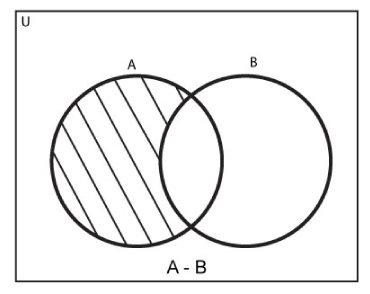# Python Set difference()

The difference() method returns the set difference of two sets.

If A and B are two sets. The set difference of A and B is a set of elements that exists only in set A but not in B. For example:

```If A = {1, 2, 3, 4}
B = {2, 3, 9}

Then,
A - B = {1, 4}
B - A = {9}```The syntax of difference() method in Python is:

`A.difference(B)`

Here, A and B are two sets. The following syntax is equivalent to `A-B`.

## Return Value from difference()

The difference() method returns the difference of two sets which is also a set. It doesn't modify original sets.

## Example 1: How difference() works in Python?

```A = {'a', 'b', 'c', 'd'}
B = {'c', 'f', 'g'}

# Equivalent to A-B
print(A.difference(B))

# Equivalent to B-A
print(B.difference(A))```

When you run the program, the output will be:

```{'b', 'a', 'd'}
{'g', 'f'}```

You can also find the set difference using - operator in Python.

## Example 2: Set Difference Using - Operator.

```A = {'a', 'b', 'c', 'd'}
B = {'c', 'f', 'g'}

print(A-B)

print(B-A)```

When you run the program, the output will be:

```{'b', 'd', 'a'}
{'f', 'g'}```